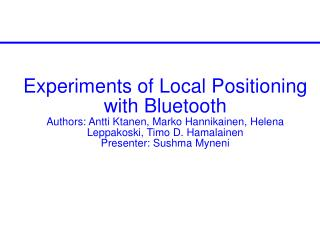DownloadDownload PresentationNovelty And Contributions

# Novelty And Contributions

Download Presentation## Novelty And Contributions

- - - - - - - - - - - - - - - - - - - - - - - - - - - E N D - - - - - - - - - - - - - - - - - - - - - - - - - - -
##### Presentation Transcript

1. Experiments of Local Positioning with BluetoothAuthors: Antti Ktanen, Marko Hannikainen, Helena Leppakoski, Timo D. HamalainenPresenter: Sushma Myneni

2. Novelty And Contributions Uses Received Power levels for estimating Distances Uses Extended Kalman Filter to compute 3D position estimates A mean absolute error of 3.76m was measured with respect to positioning the bluetooth devices

3. Local Positioning methods for Bluetooth • Angle of Arrival (AOA) • Cell Identity (CI) • Time of Arrival (TOA) • Time Difference of Arrival (TDOA) • Why are these not applicable for BT (Bluetooth) devices? • RX power levels

4. Bluetooth Overview • FHSS – 79 channels • 2.4000 – 2.4835 Ghz ISM Band • Class 1, Class 2 and Class 3 Devices

5. Bluetooth Local Positioning Application Architecture

6. Distance Measurement Based on RX Power Level • Distance Estimation using radio wave propagation model • Exchange of Transmit power information between devices

7. Introduction to Kalman filter Kalman filter is a recursive filter that estimates the state of dynamic system from a series of incomplete and noisy measurements Example Application scenario: A car which continuously provides information about its speed and current position each of which includes some error In control theory, the kalman filter is mostly referred to as Linear quadratic estimation - LQE

8. Introduction to Extended Kalman Filter EKF used when process to be estimated and measurement relationship are non-linear A kalman filter than Linearizes about the current mean and covariance is referred to as EKF

9. Extended Kalman Filter Estimates current position using the new measurement information Equation based on State dynamics Where x – 3D position of mobile device, u - Noise The equation that relates measurements to state variable is Where z is measurement function, v – Measurement noise with variance R h(x) are the distances between mobile devices and the neighbour devices Linearise the H matrix, then compute x (next step) as

10. Bluetooth module – connectivity and Measurement HCI - Computation Mobile device (Master) Stationary Neighbour devices (Slaves) with programmed positions BLPA in BT Device

11. Experimental Setup for Distance measurement Measure the distance based on RX power levels using the equation for calculating distance Significant differences between measured values and model (Mean Error - 1.41m)

12. Evaluating BPLA

13. Drawbacks Error rate very high 3.76m If correct distances is given as input to kalman filter, then error has been reduced to 0.0193m, which means than using RSSI to get RX Power Levels is not a good metric Rather than using RSSI to calculate RX power level, BT device should provide mechanism for reading the Power level information directly from the bluetooth module Experiments were conducted where there were no obstructions. What is the effect on Local positioning if interference is present? Needs another protocol layer if distribution has to be implemented.

14. Conclusion Relation to class Localization application specific Context aware migratory services – EKF? Relation to Project RSSI metric

15. Thankyou